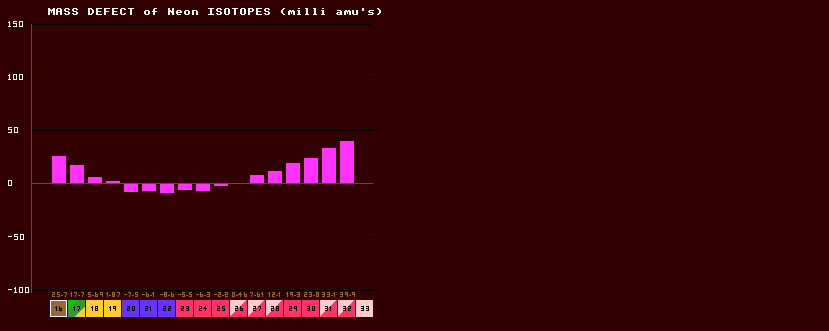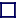### MASS DEFECT

(in amu's, atomic mass units. 1 amu=931 MeV)

 The mass of an isotope is not simply the sum of the masses of all the constituent nucleons (protons and neutrons) within the nucleus, because when they are all gathered together inside the nucleus, some of the mass is 'lost' this loss being variously and confusingly called the 'mass defect', mass decrement, mass excess and mass correction. The mass defect is simply the measured atomic mass of the isotope, M minus the mass number, A (number of neutrons plus protons) of the isotope. Because the scale is based on the atomic Mass of 12C being exactly 12.000 (by definition), most of the mass defects are negative (except 12C which is zero), with the minimum being around krypton. The lower figures (in brown) is the mass defect, given in MeV. Shown above is the Mass defect chart for the isotopes of Neon. There is absolutely no significance in the graph passing through zero (at two places in this graph); the mass defects are with reference to the mass of carbon-12 (which by definition is 12 exactly). Hence carbon-12 would have a mass defect of zero. All the graph is showing is that neon-26 has the (nearly) same mass defect as carbon-12. Similarly, neon-19 has a mass defect nearly the same as carbon-12. There is no other significance to the zero nor to the fact that neon-22 has a mass defect below zero, it is just that its' mass defect is below that of carbon-12.